Courses

# Surveying 2

## 20 Questions MCQ Test Mock test series of SSC JE Civil Engineering | Surveying 2

Description
This mock test of Surveying 2 for Civil Engineering (CE) helps you for every Civil Engineering (CE) entrance exam. This contains 20 Multiple Choice Questions for Civil Engineering (CE) Surveying 2 (mcq) to study with solutions a complete question bank. The solved questions answers in this Surveying 2 quiz give you a good mix of easy questions and tough questions. Civil Engineering (CE) students definitely take this Surveying 2 exercise for a better result in the exam. You can find other Surveying 2 extra questions, long questions & short questions for Civil Engineering (CE) on EduRev as well by searching above.
QUESTION: 1

### The horizontal angle between the true meridian and magnetic at a place is called:

Solution:

Declination: The horizontal angle between the True meridian and magnetic meridian is called declination.

Local attraction: Local attraction is the phenomenon by which the magnetic needle is constantly prevented to point towards the magnetic north at a place. This is because that these magnetic compass is influenced by other magnetic objects at that locality such as wires carrying electric current, rails, steel and iron structures, steel tapes etc.

Magnetic Bearing: The horizontal angle which a line makes with the magnetic meridian measured from Magnetic North line is called magnetic bearing. It varies with time. Magnetic meridian of a line can be measured in the field by using prismatic compass.

Azimuth: Azimuth of a line is its horizontal angle measured clockwise from geographic or true meridian.

QUESTION: 2

### Principle of plane tabling is:-

Solution:

Plane table surveying is the graphical method of survey in which the field observations and plotting are done simultaneously. Plane table surveying is based on the principle that lines drawn during plotting always lie parallel to the corresponding lines actually present on the ground i.e the principle of plane table surveying is parallelism.

QUESTION: 3

### Which of the following surveys is employed for collecting data related to transfer of land property from one owner to another?

Solution:

Cadastral survey : The cadastral survey are made incident to the fixing of property lines, the calculation of land area, or the transfer of land property from one owner to another. They are also made to fix the boundaries of municipalities and of state and federal jurisdictions.

Basically, Cadastral surveying is the discipline of land surveying that relates to the laws of land ownership and the definition of property boundaries.

Geodetic survey is the survey in which curvature of the earth is taken in to considerations in the field.

City survey: They are made in connection with the construction of streets, water supply systems, sewers and other works.

QUESTION: 4

In plane table survey, the error due to centering should not exceed the scale divided by..........

Solution:

Centring is very important for plotting detail through plane table surveying. Error in centring leads to error in plotting of location of objects. So, the operations involved in centring need careful consideration. The error due to centering should not exceed the scale divided by 40.

QUESTION: 5

The least count of a Vernier is the:

Solution:

The minimum length that can be measured using the Vernier callipers is called its least count.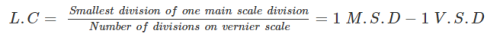QUESTION: 6

In chain surveying the lateral measurements that are taken form the chainage to the objects are called:

Solution:

Offsets: Lateral measurements to chain lines for locating ground features are known as offsets.

Backsights: A measurement or reading taken back towards a point of known elevation, used to calculate the height of the surveying instrument is known as backsights.

QUESTION: 7

The operation of levelling in which only backsight and foresight readings are taken at every step of the level is called…….levelling.

Solution:

Fly levelling is conducted when the benchmark is very far from the work station. In such case, a temporary bench mark is located at the work station which is located based on the original benchmark. Even it is not highly precise it is used for determining approximate level. In fly levelling only backsight and foresight readings are taken at every step of the level.

Reciprocal levelling: When it is not possible to locate the levelling instrument in between the inter visible points, reciprocal levelling is performed. This case appears in case of ponds or rivers etc. In case of reciprocal levelling, instrument is set nearer to 1st station and sighted towards 2nd station.

Profile leveling is generally adopted to find elevation of points along a line such as for road, rails or rivers etc. In this type of levelling, readings of intermediate stations are taken and reduced level of each station is found.

QUESTION: 8

Type of error that under same conditions, will always be of same size and sign

Solution:

Systematic errors are caused by the surveying equipment, observation methods, and certain environmental factors. Under the same measurement conditions, these errors will have the same magnitude and direction (positive or negative) because systematic errors are repetitive and tend to accumulate in a series of measurements, they are also referred to as cumulative errors.

Random (or accidental) errors are not directly related to the conditions or circumstances of the observation. For a single measurement or a series of measurements, it is the error remaining after all possible systematic errors and blunders have been eliminated.

QUESTION: 9

Formula used in correction for curvature (Cc):

where, d is the distance in km and Cc is in meter

Solution:

Curvature correction is negative and is given by:

Cc = -0.07849 d2

where,

d is the distance in km

Cc is in meter

QUESTION: 10

Which of the following is the most appropriate well conditioned traingle?

Solution:

An equilateral traingle will be the most appropriate traingle because all the angles are of 60° and have common intersection. Isosceles is also well conditioned traingle but their interior angles are may be lesser than 30° for a particular case.

The shape of the triangle in which any error in angular measurements, has a minimum effect upon the lengths of the computed sides, is known as a well conditioned triangle. Well-conditioned triangles are preferred because their apex points are very sharp and can be located by a single ‘dot’. In such a case, there is no possibility of relative displacement of the plotted point.

Condition for well conditioned triangle: angle must be in between 30° to 120°.

QUESTION: 11

If the engineer’s scale of a drawing is state as 1 cm = 5 m, then the fraction scale would be 1: x, where x is:

Solution:

Engineer's Scale is given as:

1 cm = 5 m

Now,

Representative fraction =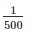Hence x = 500

QUESTION: 12

Angles of 45° with a chain line may be set out with:

Solution:

1. For 45° → French square

2. For 90° → Optical square

3. Open cross staff is commonly used for setting out long offsets

QUESTION: 13

During chaining along a straight line, the leader of the survey party has three arrows and while follower has five arrows, the distance of the follower from the starting point will be _________.

Solution:

Arrows are used for counting the number of chains while measuring a chain line. An arrow is inserted into the ground after every chain length measured on the ground. After completion of a chain the leader inserts an arrow and follower picked that one on next chaining operation. As the follower has 5 arrows so the distance between the follower and the starting point will be five chains.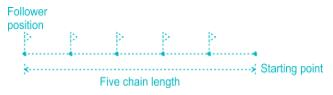QUESTION: 14

If R is radius of curvature and l is the distance from the beginning on a transition curve, then for ideal transition

Solution:

A curve of varying radius is known as transition curve. The radius of such curve varies from infinity to certain fixed value. According to requirement of ideal transition curve, the super elevation should be increased uniformly with increase of centrifugal force at a constant rate.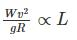Here W, v & g are constant,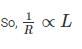QUESTION: 15

A line passing through the point of 3° declination is known as:

Solution:

Isogonic line: A line passing through points of equal declination is known as Isogonic line.

Agonic line: A line passing through the zero declination is known as Agonic line.

QUESTION: 16

As applied to staff readings, the corrections for curvature and refraction are respectively

Solution:

The curvature increases the staff reading, hence its correction is negative. The rays of light when passing through atmosphere bend down and follows a curved path, hence correction is positive.

Correction due to curvature = -0.0785D2

Correction due to refraction = 0.0112D2

Combined correction = -0.0673D2

QUESTION: 17

Which one of the following statement is CORRECT?

Solution:

In levelling, if backsight is less than foresight, then backsight station is at higher than foresight station. In reciprocal levelling, the error due to refraction, curvature is eliminated. At change point in levelling one backsight and one foresight are taken.

Due to the effect of curvature, the object is sighted to appear lower than they really are.

QUESTION: 18

Calculate the volume of the earthwork (in cubic meter) using trapezoidal method if the cross section areas of the three section of embankment at an interval of 20 m are 40 square meters, 50 square meters and 80 square meters.

Solution: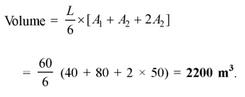QUESTION: 19

The instrument which is used in plane tabling for obtaining horizontal and vertical distance directly without resorting to changing, is known as:

Solution:

Telescopic Alidade: It consist of a telescope as an arrangement for sighting. The telescope is fitted with a stadia diaphragm and can be used as tachometer also for computations of horizontal and vertical elevations.

Planimeter: It is a measuring instrument used to determine the area of an arbitrary two dimensional shape.

Clinometer: It is an instrument for measuring angles of slope (or tilt), elevation or depression of an object with respect to gravity

QUESTION: 20

In a solution of the three-point problem in plane table surveying, the converging of error is attained through

Solution:

In three point problem, if the orientation of the plane table is not proper, the intersection of the resectors through the three points will not meet at a point but will form a triangle, known as triangle of error. The size of the triangle of error depends upon the amount of angular error in the orientation. This triangle of error will reduce to a point by trial and error.